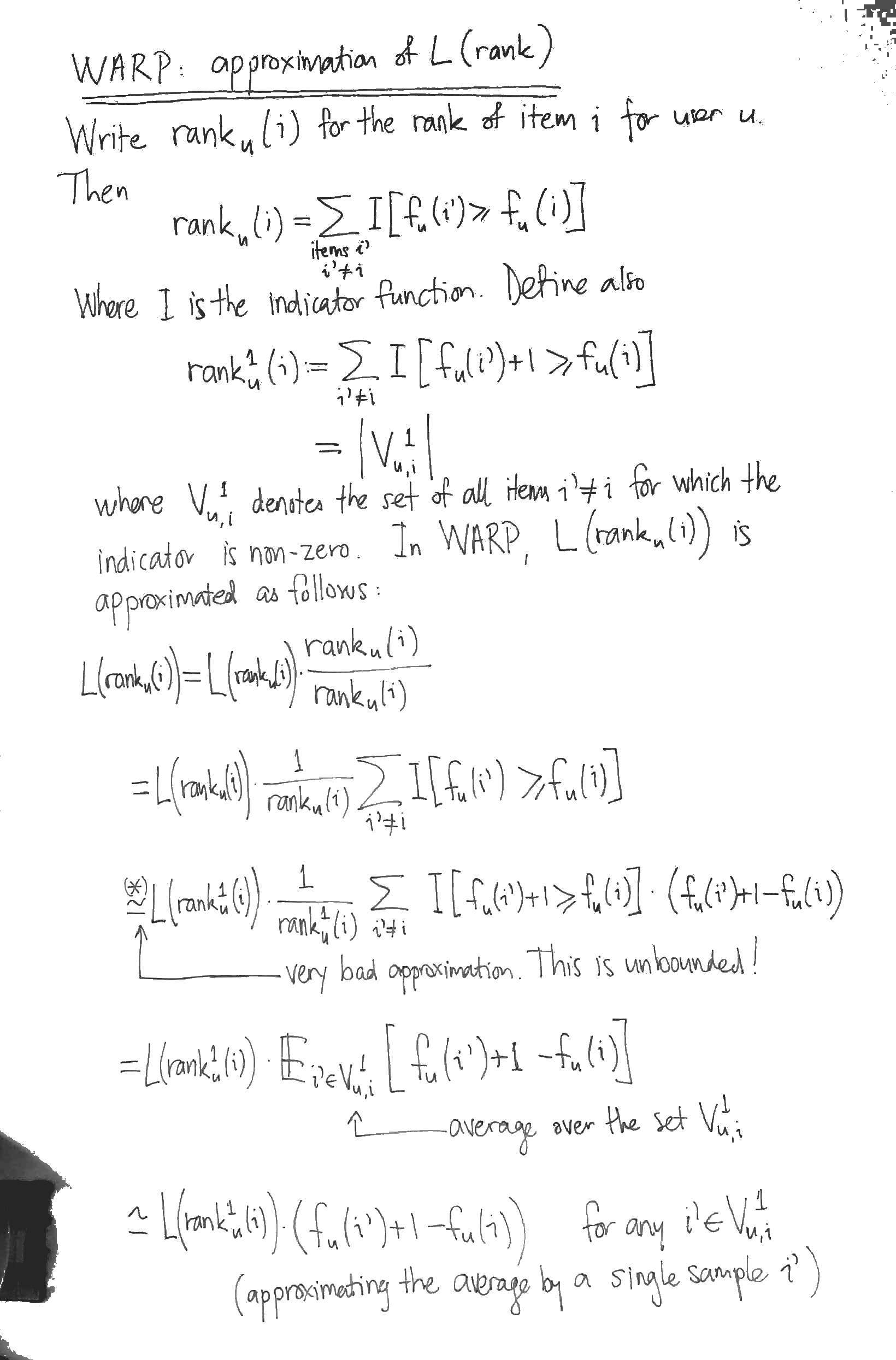# WARP loss for implicit-feedback recommendation

We consider the “Weighted Approximate-Rank Pairwise-” (WARP-) loss, as introduced in the WSABIE paper of Weston et. al (2011, see references), in the context of making recommendations using implicit feedback data, where it has been shown several times to perform excellently.  For the sake of discussion, consider the problem of recommending itemsto users, where a scoring functiongives the score of itemfor user, and the item with the highest score is recommended.

WARP considers each observed user-item interactionin turn, choses another “negative” itemthat the model believed was more appropriate to the user, and performs gradient updates to the model parameters associated to,andsuch that the models beliefs are corrected.  WARP weights the gradient updates using (a function of) the estimated rank of itemfor user.  Thus the updates are amplified if the model did not believe that the interactioncould ever occur, and are dampened if, on the other hand, if the interaction is not surprising to the model. Conveniently, the rank offorcan be estimated by counting the number of sample itemsthat had to be considered before one was found that the model (erroneously) believed more appropriate for user.

### Minimising the rank?

Ideally we would like to make updates to the model parameters that minimised the rank of itemfor user.  Previous work of Usunier (one of the authors) showed that the precision at k was best optimised when the logarithm of the rank was minimised.  (to read!)

The problem with the rank is that, while it does depend on the model parameters, this dependence is not continuous (the rank being integer valued!).  So it is not possible to speak of gradients.  So what is to be done instead?  The approach of the authors is to derive a differentiable approximation to the logarithm of the rank, and to minimise this instead.

### Derivation: approximating the (log of the) rank

WARP has been shown several times to perform very well for implicit feedback recommendation.  However, the derivation of the approximation of the log of the rank used in WARP, as outlined in the WSABIE paper, is nonsense.  I can only think that the authors arrived at WARP in another way.  Let’s look at it more closely.  In the following:

•is the score assigned by the model to itemfor user.
•is some function that defines the error as a function of the rank.  In the WSABIE paper,is approximately the natural logarithm (for the derivation below, however, it doesn’t matter whatis)The most obvious problem with the derivation is the approximation marked with an asterix (*).  At this step, the authors approximate the indication functionby.  While the latter is familiar as the hinge loss from SVMs, it is (begin unbounded!) a dreadful approximation for the indicator.  It seems to me that the sigmoid of the difference of the scores would be a much better differentiable approximation to the indicator function.

To appreciate why the derivation is nonsense, however, you have to notice that the it has nothing to do with.  That is, the derivation above would yield an approximation for, whateverhappened to be, even a constant function.

### Optimisation

WARP considers each observed interactionin turn, repeatedly sampling itemsfrom the uniform distribution over all items until it finds one in, i.e. until it finds an itemwhose score for the useris at worst 1 less than the score of the observed interaction.  For this triple, it performs gradient updates to minimise:The naive approach to computingis to calculate all the scores for the given user in order to determine the rank of the item.  WARP performs a nice trick to do much better: it estimatesby counting how many candidate negative itemsit had to consider before finding one in.  This yieldsHowever it is still the case thatis not differentiable.  So when we compute the gradients, this quantity has to be treated as a constant. Thus it simply becomes a weighting applied to the gradient of the difference of the scores (hence the name WARP, I guess).

### WARP optimises for item to user recommendations

With its negative sampling technique, WARP optimises for recommending items to a user.  For instance, the problem of recommending users to items (so, transposing the interaction matrix) is not trained for.  I wonder if some extra uplift could be obtained by training for both problems simultaneously.

### Normalising for the total number of items

With the optimisation stated as above, the learning rate will need to be re-tuned for datasets that have different numbers of items, since the gradient weightingis ranges fromto.  It would make more sense to weigh the gradient updates by:which ranges between 0 and 1.

### Implementations

There are two implementations of WARP for recommendation that I know of, both in Python:

• LightFM, written by Maciej Kula.  Works well.  Also implements BPR with uniform sampling and WARP k-OS (which I’ve not investigated yet).
• MREC, written by Levy and Jack at Mendeley, has a matrix factorisation recommender trained using WARP.  I’ve not tried this one out yet.

### References

Jason Weston, Samy Bengio and Nicolas Usunier, Wsabie: Scaling Up To Large Vocabulary Image Annotation, 2011, (PDF).